Categories

Polyrhythm II

To internalize an unfamilar polyrhythm, I suggest the following steps:
1) clap and tap the rhythm away from your instrument
2) play it on your instrument, using a single pitch (no moving notes yet)
3) if it’s a difficult passage, play it using simplified material (a simple scale pattern)
4) play as written

Here is a written-out variation on how you can practice a scalar passage 4:5.
Your first step (not shown here) could be to play repeated sixteenths (here, it would be repeated F’s) and accent every 5th one.
The first line shows how groups of 5 sixteenths fit into a 5/4 bar.
The second line fills in the ties with moving sixteenths.
The third and fourth lines are played the same but notated differently. It’s good to practice both.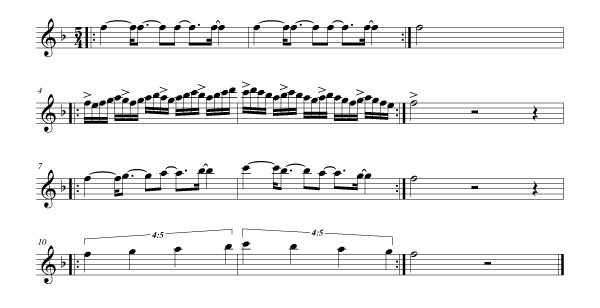When you have an even number against 3, I find it easy to feel or visualize the second half of the second beat. It serves as a goal-post, or check-point. Here is an exercise similar to that above, but with 4:3.
Remember, your first step (not shown here) could be to play repeated sixteenth F’s and accent every 3rd one.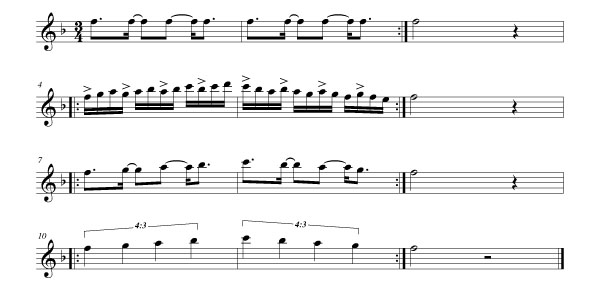Here are some further exercises with even numbers against 3.  If it helps, focus on the back of the second beat.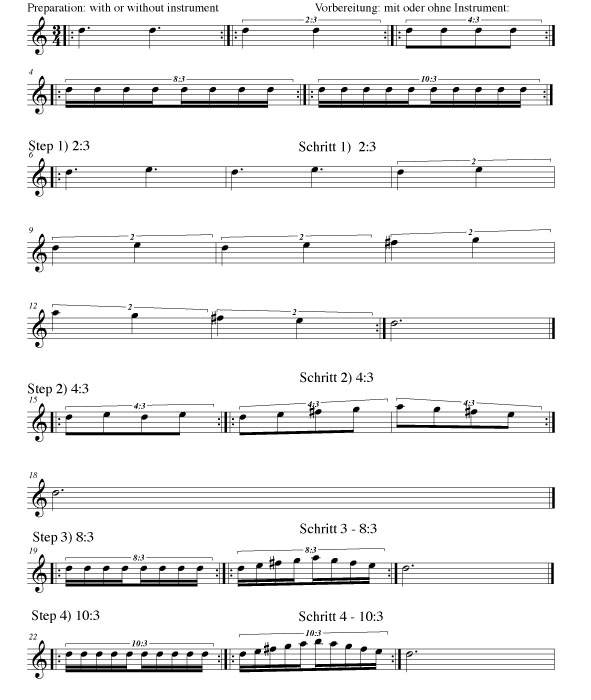In the next post, I will take the scale patterns from Taffanel/Gaubert and fit them into a polyrhythmic pattern.

Categories

Polyrhythms I

This is the first of a series about practicing complex rhythms related to a pulse, a.k.a. polyrhythms.

Why bother practicing polyrhythms? Some of us have been taught that our metronome is our best friend, but how useful is it really? Do we bother to listen to it? If we do, does it ensure us a good sense of rhythm? If some of us were honest, we would admit that we do not want to listen too closely, for fear of being labeled mechanical. After all, we want to play rhythmically, not mechanically. How to do this?

What I suggest is a method to develop rhythmical phrasing through the study of Taffanel/Gaubert’s study no. 1 from Exercices Journaliers. (I choose this because most of you reading this are flutists, and it is best to apply these ideas to something that is already familiar.). But this introduction will first cover the basics.

A well developed sense of rhythmical phrasing can help whether you want to become the next star beat-boxer or want to keep a steady Scherzo from Mendelssohn’s  A Midsummer Night’s Dream.

You will need a metronome that can be set to a simple beat pattern (2/4, 3/4, etc.), and a willingness to feel a bit clumsy at first.

The following examples use simple mathematics to see visually where your pulse is against the metronome’s (or your partner’s). It is a graphical guide to help develop a FEEL for the polyrhythm.

Given polyrhythm a:b, where b = metronome beats and a = your beats, there are two ways of figuring it out:

1) Take the b number of metronome beats and divide it into units of a, then clap or play every b number of these units. Let’s take the example where a:b = 3:2(three against two). In standard notation it looks like this: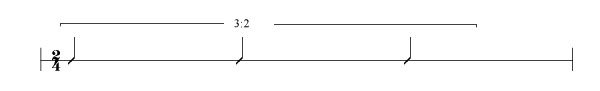Now take two (b) metronome beats, divide them into units of three (a),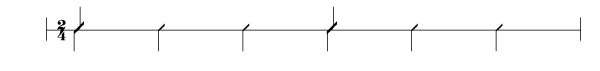Now clap or play every two (b) of these units as shown by a crossed note head: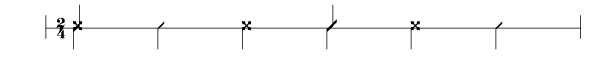_____________________________________________________________________________
2) The second way to figure a:b  is to multiply a times b, in this case 3 times 2. This gives us six:Now divide this 6 into a (3). That gives us two, so mark every two pulses with an O on top. Then divide 6 into b(2) . This gives us 3, so marks every 3 pulses with an X on the bottom.The “O”s are the rhythm you play or clap, the “X”s are the metronome beats.

Here is a more complicated example: 5:7Let’s see how it looks using the first method: given a:b, divide b (7) into units of a (5) :Now clap or play every b of these units (7) notated by a crossed note head:Try writing this example using the second method. (Begin by multiplying 5 x 7.)
The next installation will give practical exercises on how to feel 5:4, and any even number against 3.
For more practice, see Peter Magadini’s Polyrhythms, the Musician’s Guide, Hal Leonard Publications, 1993
Categories

Tips for Complex Rhythms à la Ferneyhough’s Superscriptio

Below is some advice for works by composers (such as Brian Ferneyhough) who use complex rhythms not based on pulse-centered activity. I spoke with Mr. Ferneyhough about this subject and he was very clear that in his music, the measure is a “domain of a certain energy quotient” not related to a pulse.

In other words, a measure can be interpreted as an “area of activity”. The level of activity can easily be reckoned using the starting tempo. From there we can calculate the length of any measure or individual note through simple mathematics.

So if the rhythm cannot be felt as a pulse, one can at least memorize the speed in which it is supposed to happen. Surprisingly, it’s sometimes much slower than one thinks!

Ferneyhough’s Superscriptio for solo piccolo is a good example. The basic tempo is an eighth-note at 56. This means a whole note in 4/4 equals 7, because there are 8 eighth notes in a 4/4 bar, and 8 divided by 56 = 7.

From this number 7 you can deduce all the “odd” time signatures that are not based on divisions of the eighth note. An eighth note quintuplet (or a “1/10” bar) will equal 70 because there are 10 quintuplets in a 4/4 bar (7 x 10 = 70). To find the length of a 3/10 bar you would divide 70 by 3. If you have a 5/10 bar you would divide 70 by 5. An eighth note triplet (or a “1/12” bar) will equal 84 because there are 12 triplets in a 4/4 bar (7 x 12 = 84). To find the length of a 3/12 bar you would divide 84 by 3, and to find the length of a 5/12 bar you would divide 84 by 5.

What if the compound rhythms are stacked on top of each other, as in Ferneyhough’s other works? This is an imaginary example:Let’s imagine as in Superscriptio the starting tempo is an eighth-note at 56. The last six 16ths (at the end of the bar) roughly equal two 16th-note triplets at 64. The nine 32nds under the 9:5 are roughly equal to three sets of 32nd triplets going at 116.

It could have been notated thus (among other possibilities):Here’s the math:
From above we know that a 1/10 bar equals 70.
A 4/10 bar will equal 17.5 (70 divided by 4 = 17.5).
Each 11-tuplet will be 192.5 (17.5 x 11 = 192.5).
There are 6 of these 11-tuplets at the end. If we think of them as two 16th-note triplets, divide 192.5 by 3 = ca. 64

5 of those 11-tuplets equals 38.5 (192.5 divided by 5 = 38.5).
In the 9:5, if you think of the 9-tuplets as 3 sets of 32nd triplets, those triplets go at ca. 116 (38.5 x 3 = 115.5)

A click track will ensure accuracy without a doubt. However, if you opt for another approach, try the following: memorize the speed of each bar by practicing related bars together. Keeping Superscriptio as an example, you can practice all the bars based on “1/12” while keeping the metronome at tempo 84. (Yes, you will be jumping from measure to measure, or page to page.) Then do the same for all the “1/10” bars, then “1/8” etc. You are not trying to achieve musical continuity yet, this is just an exercise to help relate all the bars with this tempo, and to keep them consistent. When you finally put the piece together, your “internal conductor” will hopefully have a kinesthetic memory of the pulse of each measure and make the tempo changes accordingly.

Also see my post Seminar with Brian Ferneyhough.
Other thoughts?